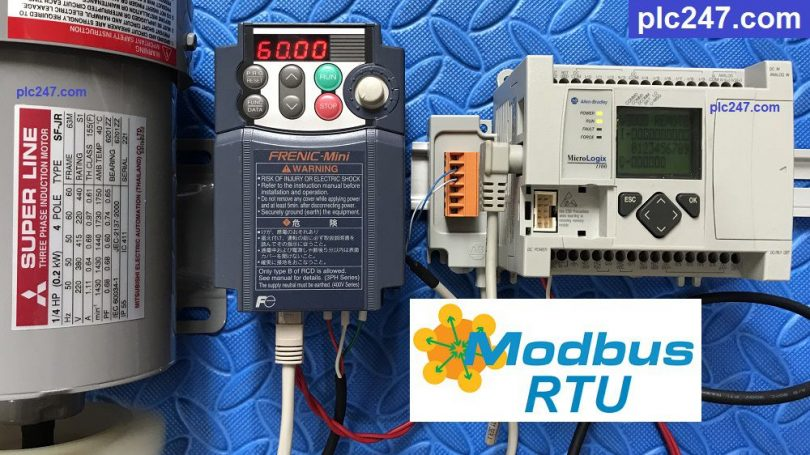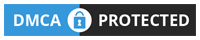# Micrologix 1100 “Modbus RTU” FUJI Frenic VFDWritten by

Hello everyone. Today plc247.com would like to write a tutorial on how to connect PLC MicroLogix with FUJI Frenic Series inverter via Modbus-RTU RS485 Communication.

The PLC model I use is MicroLogix 1100 and FUJI Frenic Mini inverter, other FUJI Frenic Series do the same (Frenic Mini, Frenic Eco, Frenic Multi, Frenic MEGA)

#### FUJI Frenic Modbus RTU Configuration

In order for the inverter to run in Modbus-RS485 mode, we need to set the following parameters:

• H30 = 3 (VFD Control via RS485 Port)
• Y01 = 1 (Slave Address)
• Y02 = 0
• Y03 = 2
• Y04 = 2 (9600bps)
• Y05 = 0 (data: 8bit)
• Y06 = 1 (parity check: Even)
• Y07 = 1 (stop bit: 1)
• Y10 = 0 (Modbus RTU Selection)
• Y99 = 0
##### FUJI Frenic Modbus Address Table

Below is a table of hex addresses of different Command Groups

• Frequency Reference = S (07H) + 05 = 705 (hex)
>>> PLC Address = 705 (hex) + 1 = 1797 (dec) + 1 = 1798
• Motor Control = S (07H) + 06 = 706 (hex)
>>> PLC Address = 706 (hex) + 1 = 1798 (dec) + 1 = 1799

+ Set Word 1799 = 1 >>> Forward Run
= 2 >>> Reverse Run
= 0 >>> Stop

In this project plc247.com reads 4 data feedback registers from the inverter consecutively, starting from registers M09 > M12

• Output Frequency = M (08H) + 09 = 809 (hex)
>>> PLC Address = 809 (hex) + 1 = 2057(dec) +1 = 2058

### MicroLogix 1100 Programming

+ MicroLogix 1100 Channel Configuration

+ Operation Control Command

+ Frequency Setting Command

### Detailed Video Tutorial

======

Document